1. Math Worksheets>
2. Statistics>
3. Stem and Leaf Plot

# Stem-and-leaf Plot Worksheets

Stem-and-leaf plot worksheets contain a set of data that are to be ordered and to be presented in a stem and leaf plot. The data are to be interpreted and questions based on it are to be answered in the make and interpret plot pages. Stem-and-leaf plots also contain back-to-back plots, rounding data, truncating data and more.

Make a stem-and-leaf plot: Level 1

Exclusive six sets of data are given in these pages. Order the data and draw stem-and-leaf plots. Also fill in the key.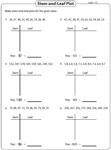Make a stem-and-leaf plot: Level 2

Every worksheet has four sets of data that are to be sequenced and to be represented in stem-and-leaf plots.Make a stem-and-leaf plot: Scenario

Each sheet has two scenarios given. Around 15 to 20 data are given in each question. Order the given data to make stem-and-leaf plots.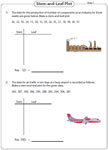Read the stem-and-leaf plot for each scenario and interpret the data to answer questions on mode, range, maximum and minimum values.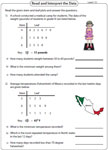Each sheet has two scenarios with word problems. Answer the questions on mode, range, maximum, minimum, median and average in these sheets.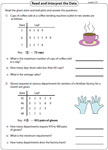Make and interpret: Level 1

Each worksheet has a scenario and a set of data based on it. Make a stem-and-leaf plot for the given data and answer the questions.Make and interpret: Level 2

For each scenario, there are five standard word problems given. Make a stem-and-leaf plot and analyze the data to answer the questions.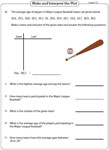Making back-to-back stem-and-leaf plot

Two sets of data are given for each question. Use the data and make three back-to-back stem-and-leaf plots in each worksheet. Also write the key.Interpreting back-to-back stem-and-leaf plot

Interpreting back-to-back stem-and-leaf plots have three exclusive worksheets with two sets of data. Make back-to-back plots and answer the questions.Round and make a stem-leaf plot

In each sheet three sets of data are given. Round each whole / decimal number to the nearest ten, hundred or tenths. Make stem-and-leaf plots.Truncate and make a stem-leaf plot

Truncate each number from the given data to the nearest two-digit or three-digit. Make a stem-and-leaf plot and specify the key range.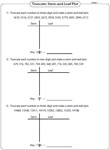Obtain the entire collection of worksheets on stem-and-leaf plot in a jiffy!

Related Worksheets